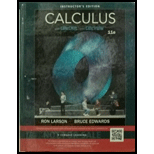Chapter 2.4, Problem 3E### Calculus (MindTap Course List)

11th Edition
Ron Larson + 1 other
ISBN: 9781337275347

#### Solutions

Chapter
Section### Calculus (MindTap Course List)

11th Edition
Ron Larson + 1 other
ISBN: 9781337275347
Textbook Problem

# Decomposition of a Composite Function In Exercises 3-8, complete the table. y = f ( g ( x ) ) u = g ( x ) y = f ( u ) y = ( 6 x − 5 ) 4

To determine

To calculate: The values of u=g(x) and y=f(u) of the function expressed as, f(g(x))=(6x5)4.

Explanation

Given:

The function is given to be, y=(6x5)4.

Calculation:

The given function is expressed as,

y=(6x5)4

In order to find the derivative of the function, consider u=6x5

### Still sussing out bartleby?

Check out a sample textbook solution.

See a sample solution

#### The Solution to Your Study Problems

Bartleby provides explanations to thousands of textbook problems written by our experts, many with advanced degrees!

Get Started

## Additional Math Solutions

#### Expand each expression in Exercises 122. (x+1)(x3)

Finite Mathematics and Applied Calculus (MindTap Course List)

#### In Exercises 722, evaluate the expression. 21. 161/4 81/3

Applied Calculus for the Managerial, Life, and Social Sciences: A Brief Approach

#### Write the following numbers in word form. .000033

Contemporary Mathematics for Business & Consumers

#### Differentiate the function. y = log2 (x log5 x)

Single Variable Calculus: Early Transcendentals